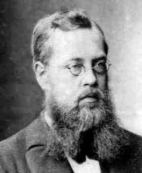A# Lie, Marius Sophus (1842–1899)Sophus Lie was a Norwegian mathematician who, along with his close friend Felix Klein, introduced group theory into geometry and used it to classify geometries. Lie discovered the contact transformation, which maps curves into surfaces (1870), and Lie groups, which use continuous or infinitesimal transformations. He used these groups to classify partial differential equations, making the traditional methods of solution all reduce to a single principle. Lie groups also provided a basis for the growth of modern topology.

## Lie algebra

Lie algebra is an algebra in which multiplication satisfies properties similar to the so-called bracket operation on matrices given by [A, B] = ABBA, where the operation on the right-hand side involves ordinary multiplication and subtraction of matrices. The operation is not associative.